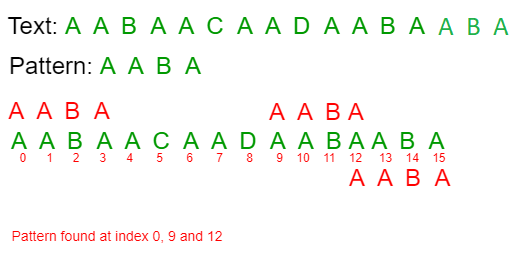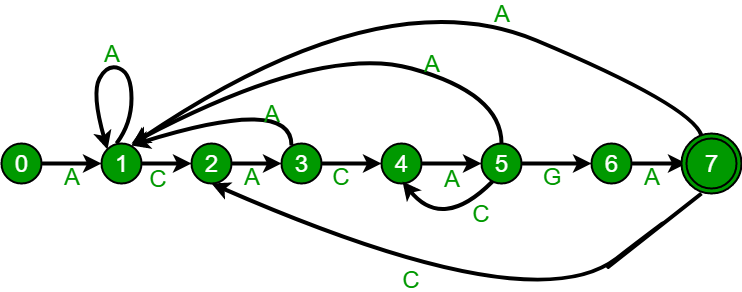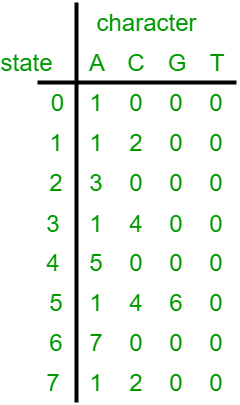Open In App
Related Articles

# Finite Automata algorithm for Pattern Searching

Given a text txt[0..n-1] and a pattern pat[0..m-1], write a function search(char pat[], char txt[]) that prints all occurrences of pat[] in txt[]. You may assume that n > m.
Examples:

```Input:  txt[] = "THIS IS A TEST TEXT"
pat[] = "TEST"
Output: Pattern found at index 10

pat[] =  "AABA"
Output: Pattern found at index 0
Pattern found at index 9
Pattern found at index 12```Pattern searching is an important problem in computer science. When we do search for a string in notepad/word file or browser or database, pattern searching algorithms are used to show the search results.

The string-matching automaton is a very useful tool which is used in string matching algorithm.
String matching algorithms build a finite automaton scans the text string T for all occurrences of the pattern P.

FINITE AUTOMATA

• Idea of this approach is to build finite automata to scan text T for finding all occurrences of pattern P.
•  This approach examines each character of text exactly once to find the pattern. Thus it takes linear time for matching but preprocessing time may be large.
• It is defined by tuple M = {Q, Σ, q, F, d} Where Q = Set of States in finite automata

Σ=Sets of input symbols

q. = Initial state

F = Final State

σ = Transition function

• Time Complexity = O(M³|Σ|)

A finite automaton M is a 5-tuple (Q, q0,A,∑δ), where

Q is a finite set of states,
q0 ∈ Q is the start state,
A ⊆ Q is a notable set of accepting states,
is a finite input alphabet,
δ is a function from Q x ∑ into Q called the transition function of M.
The finite automaton starts in state q0 and reads the characters of its input string one at a time. If the automaton is in state q and reads input character a, it moves from state q to state δ (q, a). Whenever its current state q is a member of A, the machine M has accepted the string read so far. An input that is not allowed is rejected.

A finite automaton M induces a function ∅ called the called the final-state function, from ∑* to Q such that ∅(w) is the state M ends up in after scanning the string w. Thus, M accepts a string w if and only if ∅(w) ∈ A.

## Algorithm-

```FINITE AUTOMATA (T, P)
State <- 0
for l <- 1 to n
State <- δ(State, ti)
If State == m then
Match Found
end
end```

Why it is efficient?

These string matching automaton are very efficient because they examine each text character exactly once, taking constant time per text character. The matching time used is O(n) where n is the length of Text string.

But the preprocessing time i.e. the time taken to build the finite automaton can be large if ∑ is large.

Before we discuss Finite Automaton construction, let us take a look at the following Finite Automaton for pattern ACACAGA.The above diagrams represent graphical and tabular representations of pattern ACACAGA.

Number of states in Finite Automaton will be M+1 where M is length of the pattern. The main thing to construct Finite Automaton is to get the next state from the current state for every possible character.

Given a character x and a state k, we can get the next state by considering the string “pat[0..k-1]x” which is basically concatenation of pattern characters pat, pat …pat[k-1] and the character x. The idea is to get length of the longest prefix of the given pattern such that the prefix is also suffix of “pat[0..k-1]x”. The value of length gives us the next state.

For example, let us see how to get the next state from current state 5 and character ‘C’ in the above diagram. We need to consider the string, “pat[0..4]C” which is “ACACAC”. The length of the longest prefix of the pattern such that the prefix is suffix of “ACACAC”is 4 (“ACAC”). So the next state (from state 5) is 4 for character ‘C’.

In the following code, computeTF() constructs the Finite Automaton. The time complexity of the computeTF() is O(m^3*NO_OF_CHARS) where m is length of the pattern and NO_OF_CHARS is size of alphabet (total number of possible characters in pattern and text). The implementation tries all possible prefixes starting from the longest possible that can be a suffix of “pat[0..k-1]x”. There are better implementations to construct Finite Automaton in O(m*NO_OF_CHARS) (Hint: we can use something like lps array construction in KMP algorithm).

We have covered the better implementation in our next post on pattern searching.

## C

 `// C program for Finite Automata Pattern searching``// Algorithm``#include``#include``#define NO_OF_CHARS 256` `int` `getNextState(``char` `*pat, ``int` `M, ``int` `state, ``int` `x)``{``    ``// If the character c is same as next character``    ``// in pattern,then simply increment state``    ``if` `(state < M && x == pat[state])``        ``return` `state+1;` `    ``// ns stores the result which is next state``    ``int` `ns, i;` `    ``// ns finally contains the longest prefix``    ``// which is also suffix in "pat[0..state-1]c"` `    ``// Start from the largest possible value``    ``// and stop when you find a prefix which``    ``// is also suffix``    ``for` `(ns = state; ns > 0; ns--)``    ``{``        ``if` `(pat[ns-1] == x)``        ``{``            ``for` `(i = 0; i < ns-1; i++)``                ``if` `(pat[i] != pat[state-ns+1+i])``                    ``break``;``            ``if` `(i == ns-1)``                ``return` `ns;``        ``}``    ``}` `    ``return` `0;``}` `/* This function builds the TF table which represents4``    ``Finite Automata for a given pattern */``void` `computeTF(``char` `*pat, ``int` `M, ``int` `TF[][NO_OF_CHARS])``{``    ``int` `state, x;``    ``for` `(state = 0; state <= M; ++state)``        ``for` `(x = 0; x < NO_OF_CHARS; ++x)``            ``TF[state][x] = getNextState(pat, M, state, x);``}` `/* Prints all occurrences of pat in txt */``void` `search(``char` `*pat, ``char` `*txt)``{``    ``int` `M = ``strlen``(pat);``    ``int` `N = ``strlen``(txt);` `    ``int` `TF[M+1][NO_OF_CHARS];` `    ``computeTF(pat, M, TF);` `    ``// Process txt over FA.``    ``int` `i, state=0;``    ``for` `(i = 0; i < N; i++)``    ``{``        ``state = TF[state][txt[i]];``        ``if` `(state == M)``            ``printf` `(``"\n Pattern found at index %d"``,``                                           ``i-M+1);``    ``}``}` `// Driver program to test above function``int` `main()``{``    ``char` `*txt = ``"AABAACAADAABAAABAA"``;``    ``char` `*pat = ``"AABA"``;``    ``search(pat, txt);``    ``return` `0;``}`

## CPP

 `// CPP program for Finite Automata Pattern searching``// Algorithm``#include ``using` `namespace` `std;``#define NO_OF_CHARS 256` `int` `getNextState(string pat, ``int` `M, ``int` `state, ``int` `x)``{``    ``// If the character c is same as next character``    ``// in pattern,then simply increment state``    ``if` `(state < M && x == pat[state])``        ``return` `state+1;` `    ``// ns stores the result which is next state``    ``int` `ns, i;` `    ``// ns finally contains the longest prefix``    ``// which is also suffix in "pat[0..state-1]c"` `    ``// Start from the largest possible value``    ``// and stop when you find a prefix which``    ``// is also suffix``    ``for` `(ns = state; ns > 0; ns--)``    ``{``        ``if` `(pat[ns-1] == x)``        ``{``            ``for` `(i = 0; i < ns-1; i++)``                ``if` `(pat[i] != pat[state-ns+1+i])``                    ``break``;``            ``if` `(i == ns-1)``                ``return` `ns;``        ``}``    ``}` `    ``return` `0;``}` `/* This function builds the TF table which represents4``    ``Finite Automata for a given pattern */``void` `computeTF(string pat, ``int` `M, ``int` `TF[][NO_OF_CHARS])``{``    ``int` `state, x;``    ``for` `(state = 0; state <= M; ++state)``        ``for` `(x = 0; x < NO_OF_CHARS; ++x)``            ``TF[state][x] = getNextState(pat, M, state, x);``}` `/* Prints all occurrences of pat in txt */``void` `search(string pat, string txt)``{``    ``int` `M = pat.size();``    ``int` `N = txt.size();` `    ``int` `TF[M+1][NO_OF_CHARS];` `    ``computeTF(pat, M, TF);` `    ``// Process txt over FA.``    ``int` `i, state=0;``    ``for` `(i = 0; i < N; i++)``    ``{``        ``state = TF[state][txt[i]];``        ``if` `(state == M)``            ``cout<<``" Pattern found at index "``<< i-M+1<

## Java

 `// Java program for Finite Automata Pattern``// searching Algorithm``class` `GFG {``    ` `    ``static` `int` `NO_OF_CHARS = ``256``;``    ``static` `int` `getNextState(``char``[] pat, ``int` `M, ``                             ``int` `state, ``int` `x)``    ``{``        ` `        ``// If the character c is same as next``        ``// character in pattern,then simply``        ``// increment state``        ``if``(state < M && x == pat[state])``            ``return` `state + ``1``;``            ` `        ``// ns stores the result which is next state``        ``int` `ns, i;` `        ``// ns finally contains the longest prefix``        ``// which is also suffix in "pat[0..state-1]c"` `        ``// Start from the largest possible value``        ``// and stop when you find a prefix which``        ``// is also suffix``        ``for` `(ns = state; ns > ``0``; ns--)``        ``{``            ``if` `(pat[ns-``1``] == x)``            ``{``                ``for` `(i = ``0``; i < ns-``1``; i++)``                    ``if` `(pat[i] != pat[state-ns+``1``+i])``                        ``break``;``                    ``if` `(i == ns-``1``)``                        ``return` `ns;``            ``}``        ``}` `            ``return` `0``;``    ``}` `    ``/* This function builds the TF table which``    ``represents Finite Automata for a given pattern */``    ``static` `void` `computeTF(``char``[] pat, ``int` `M, ``int` `TF[][])``    ``{``        ``int` `state, x;``        ``for` `(state = ``0``; state <= M; ++state)``            ``for` `(x = ``0``; x < NO_OF_CHARS; ++x)``                ``TF[state][x] = getNextState(pat, M, state, x);``    ``}` `    ``/* Prints all occurrences of pat in txt */``    ``static` `void` `search(``char``[] pat, ``char``[] txt)``    ``{``        ``int` `M = pat.length;``        ``int` `N = txt.length;` `        ``int``[][] TF = ``new` `int``[M+``1``][NO_OF_CHARS];` `        ``computeTF(pat, M, TF);` `        ``// Process txt over FA.``        ``int` `i, state = ``0``;``        ``for` `(i = ``0``; i < N; i++)``        ``{``            ``state = TF[state][txt[i]];``            ``if` `(state == M)``                ``System.out.println(``"Pattern found "``                          ``+ ``"at index "` `+ (i-M+``1``));``        ``}``    ``}` `    ``// Driver code``    ``public` `static` `void` `main(String[] args)``    ``{``        ``char``[] pat = ``"AABAACAADAABAAABAA"``.toCharArray();``        ``char``[] txt = ``"AABA"``.toCharArray();``        ``search(txt,pat);``    ``}``}` `// This code is contributed by debjitdbb.`

## Python3

 `# Python program for Finite Automata``# Pattern searching Algorithm` `NO_OF_CHARS ``=` `256` `def` `getNextState(pat, M, state, x):``    ``'''``    ``calculate the next state``    ``'''` `    ``# If the character c is same as next character``      ``# in pattern, then simply increment state` `    ``if` `state < M ``and` `x ``=``=` `ord``(pat[state]):``        ``return` `state``+``1` `    ``i``=``0``    ``# ns stores the result which is next state` `    ``# ns finally contains the longest prefix``     ``# which is also suffix in "pat[0..state-1]c"` `     ``# Start from the largest possible value and``      ``# stop when you find a prefix which is also suffix``    ``for` `ns ``in` `range``(state,``0``,``-``1``):``        ``if` `ord``(pat[ns``-``1``]) ``=``=` `x:``            ``while``(i

## C#

 `// C# program for Finite Automata Pattern``// searching Algorithm``using` `System;` `class` `GFG``{` `public` `static` `int` `NO_OF_CHARS = 256;``public` `static` `int` `getNextState(``char``[] pat, ``int` `M,``                               ``int` `state, ``int` `x)``{` `    ``// If the character c is same as next``    ``// character in pattern,then simply``    ``// increment state``    ``if` `(state < M && (``char``)x == pat[state])``    ``{``        ``return` `state + 1;``    ``}` `    ``// ns stores the result``    ``// which is next state``    ``int` `ns, i;` `    ``// ns finally contains the longest``    ``// prefix which is also suffix in``    ``// "pat[0..state-1]c"` `    ``// Start from the largest possible ``    ``// value and stop when you find a``    ``// prefix which is also suffix``    ``for` `(ns = state; ns > 0; ns--)``    ``{``        ``if` `(pat[ns - 1] == (``char``)x)``        ``{``            ``for` `(i = 0; i < ns - 1; i++)``            ``{``                ``if` `(pat[i] != pat[state - ns + 1 + i])``                ``{``                    ``break``;``                ``}``            ``}``                ``if` `(i == ns - 1)``                ``{``                    ``return` `ns;``                ``}``        ``}``    ``}` `        ``return` `0;``}` `/* This function builds the TF table which``represents Finite Automata for a given pattern */``public` `static` `void` `computeTF(``char``[] pat,``                             ``int` `M, ``int``[][] TF)``{``    ``int` `state, x;``    ``for` `(state = 0; state <= M; ++state)``    ``{``        ``for` `(x = 0; x < NO_OF_CHARS; ++x)``        ``{``            ``TF[state][x] = getNextState(pat, M,``                                        ``state, x);``        ``}``    ``}``}` `/* Prints all occurrences of``   ``pat in txt */``public` `static` `void` `search(``char``[] pat,``                          ``char``[] txt)``{``    ``int` `M = pat.Length;``    ``int` `N = txt.Length;`  `    ``int``[][] TF = RectangularArrays.ReturnRectangularIntArray(M + 1,``                                                      ``NO_OF_CHARS);` `    ``computeTF(pat, M, TF);` `    ``// Process txt over FA.``    ``int` `i, state = 0;``    ``for` `(i = 0; i < N; i++)``    ``{``        ``state = TF[state][txt[i]];``        ``if` `(state == M)``        ``{``            ``Console.WriteLine(``"Pattern found "` `+``                              ``"at index "` `+ (i - M + 1));``        ``}``    ``}``}` `public` `static` `class` `RectangularArrays``{``public` `static` `int``[][] ReturnRectangularIntArray(``int` `size1,``                                                ``int` `size2)``{``    ``int``[][] newArray = ``new` `int``[size1][];``    ``for` `(``int` `array1 = 0; array1 < size1; array1++)``    ``{``        ``newArray[array1] = ``new` `int``[size2];``    ``}` `    ``return` `newArray;``}``}`  `// Driver code``public` `static` `void` `Main(``string``[] args)``{``    ``char``[] pat = ``"AABAACAADAABAAABAA"``.ToCharArray();``    ``char``[] txt = ``"AABA"``.ToCharArray();``    ``search(txt,pat);``}``}` `// This code is contributed by Shrikant13`

## Javascript

 ``

Output:

```  Pattern found at index 0
Pattern found at index 9
Pattern found at index 13```

Time Complexity: O(m2)
Auxiliary Space: O(m)

References:
Introduction to Algorithms by Thomas H. Cormen, Charles E. Leiserson, Ronald L. Rivest, Clifford Stein